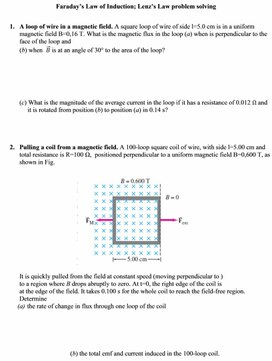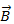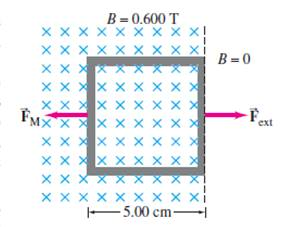4Lenz rule, phenomenon of self-inductance
Оценка 4.7

# 4Lenz rule, phenomenon of self-inductance

Оценка 4.7
docx
07.05.20204Lenz rule, phenomenon of self-inductance.docx

Faraday’s Law of Induction; Lenz’s Law problem solving

1.      A loop of wire in a magnetic field. A square loop of wire of side l=5.0 cm is in a uniform magnetic field B=0,16 T. What is the magnetic flux in the loop (a) when is perpendicular to the face of the loop and

(b) whenis at an angle of 30° to the area of the loop?

(c) What is the magnitude of the average current in the loop if it has a resistance of 0.012 Ω and

it is rotated from position (b) to position (a) in 0.14 s?

2.      Pulling a coil from a magnetic field. A 100-loop square coil of wire, with side l=5.00 cm and total resistance is R=100 Ω,  positioned perpendicular to a uniform magnetic field B=0,600 T, as shown in Fig.It is quickly pulled from the field at constant speed (moving perpendicular to )

to a region where B drops abruptly to zero. At t=0, the right edge of the coil is

at the edge of the field. It takes 0.100 s for the whole coil to reach the field-free region.

Determine

(a)   the rate of change in flux through one loop of the coil

(b) the total emf and current induced in the 100-loop coil.

Скачать файл